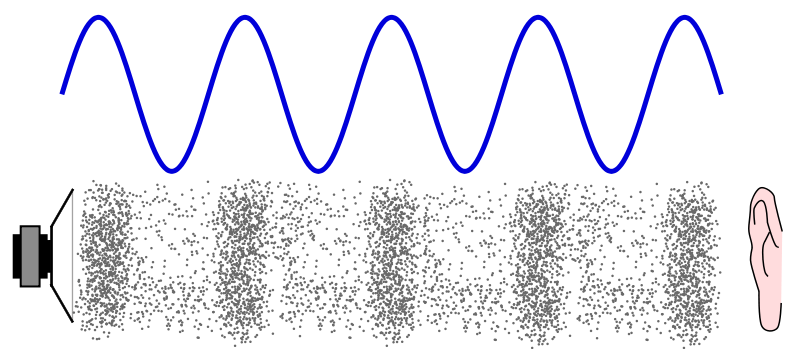# Representing Sound

## Sound Waves

Analogue vs Digital

When sound is created, a continuously changing wave of vibrations travels through the air towards our ears, where we are able to ‘hear’ it.

This wave is usually represented by a wiggly line like this:The height of the wiggly line represents how loud the sound is and is called the amplitude. The bigger the amplitude (i.e. the taller the wave), the louder the sound is.

The number of waves that occur in a period of time is called the frequency. The higher the frequency (i.e. the closer together the waves are), the higher pitch the sound is.

Sound, when it is produced, is referred to as an analogue signal. This means that it is constantly changing. However, a computer isn’t able to process this, and the signal needs to be turned into a digital signal of discrete (binary) values. This is done using an analogue to digital converter.

What is the word used to describe how loud a sound is?
amplitude
What is the word used to describe the pitch of a sound?
frequency

## Sampling

Sampling is the process whereby an analogue wave is converted into a digital signal. At specific intervals of time, measurements of the amplitude of the wave are taken and stored as a binary value.

The number of these samples taken each second is known as the Sample Rate, and is measured in Hertz (Hz). CDs are usually sampled at a sample rate of 44,100 Hz, which means 44,100 measurements of amplitude are taken of the sound wave every second.

When the analogue wave is converted using sampling, the resulting digital signal is not identical to the original. Some information is lost between each of the samples taken, and the sound loses some of its quality. By decreasing the gap between the samples (increasing the sample rate), we lose less data so the more accurate the digital recording is of the original sound wave.

The other thing that determines the quality of a recording is the Sample Resolution. This is the number of bits used to store each measurement of amplitude. For example, a sample resolution of just 2 bits, would mean that only 4 different measurements of amplitude could be recorded, and the sound is likely to be very poor quality.

CD quality for music recording is 16 bits, which means over 65,000 possible measurements of amplitude. Although, the recommendation is to record it using 24 bits to gather over 16 million possible measurements of amplitude.What is the sample rate measured in?

## File Size

As with images, just knowing a few key pieces of information will allow you to calculate what the minimum file size is of a sound recording:

File size (bits) = sampling rate x resolution x time in seconds

For example, a 3-minute (180 seconds) long song with a sample rate of 44,100 Hz, and a resolution of 24 bits would take up at least:

44100 x 24 x 180 = 190,512,000 bits = 23,814,000 bytes = 23.814 MB

As with images though, this is just the minimum file size, as there is always metadata stored with the sound file, which allows the computer to know what the sample rate and resolution is to allow it to play the sound more accurately. This increases the file size in general.

What is the minimum size of this file in bits?2 minute 30 second song recorded at 44,100 Hz and 16-bit resolution?
105840000
What is the minimum size of this file in bits?1 minute 45 second film soundtrack recorded at 48,000 Hz and 24-bit resolution?
120960000